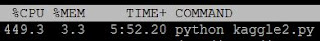Friday, November 13, 2015

Using Google's TensorFlow for Kaggle competition

Recently, Google Brain team released their neural network library 'TensorFlow'. Since Google has a state-of-the-art Deep Learning system, I wanted to explore TensorFlow by trying it out for my first Kaggle submission (Digit Recognition) . After spending few hours getting to know their jargons/APIs, I modified their multilayer convolution neural network and came 140th (with 98.4% accuracy) ... not too shabby for my first submission :D.

If you are interested in outscoring me, apply cross-validation on the below python code or may be consider using ensembles:
from input_data import *
import pandas

class DataSets(object):
pass

mnist = DataSets()

train_images = numpy.multiply(df.drop('label', 1).values, 1.0 / 255.0)
train_labels = dense_to_one_hot(df['label'].values)

#Add MNIST data from Yan LeCun's website for better accuracy. We hold out test, just for accuracy sake, but could have easily added it :)

mnist2 = read_data_sets("/tmp/data/", one_hot=True)
train_images = numpy.concatenate((train_images, mnist2.train._images), axis=0)
train_labels = numpy.concatenate((train_labels, mnist2.train._labels), axis=0)

VALIDATION_SIZE = 5000
validation_images = train_images[:VALIDATION_SIZE]
validation_labels = train_labels[:VALIDATION_SIZE]
train_images = train_images[VALIDATION_SIZE:]
train_labels = train_labels[VALIDATION_SIZE:]

mnist.train = DataSet([], [], fake_data=True)
mnist.train._images = train_images
mnist.train._labels = train_labels

mnist.validation = DataSet([], [], fake_data=True)
mnist.validation._images = validation_images
mnist.validation._labels = validation_labels

test_images = numpy.multiply(df1.values, 1.0 / 255.0)
numTest = df1.shape
test_labels = dense_to_one_hot(numpy.repeat(, numTest))
mnist.test = DataSet([], [], fake_data=True)
mnist.test._images = test_images
mnist.test._labels = test_labels

import tensorflow as tf
sess = tf.InteractiveSession()
x = tf.placeholder("float", [None, 784])   # x is input features
W = tf.Variable(tf.zeros([784,10]))           # weights
b = tf.Variable(tf.zeros())                    # bias
y_ = tf.placeholder("float", [None,10])   # y' is input labels
#Weight Initialization
def weight_variable(shape):
initial = tf.truncated_normal(shape, stddev=0.1)
return tf.Variable(initial)

def bias_variable(shape):
initial = tf.constant(0.1, shape=shape)
return tf.Variable(initial)

# Convolution and Pooling
def conv2d(x, W):
return tf.nn.conv2d(x, W, strides=[1, 1, 1, 1], padding='SAME')

def max_pool_2x2(x):
return tf.nn.max_pool(x, ksize=[1, 2, 2, 1], strides=[1, 2, 2, 1], padding='SAME')

# First Convolutional Layer
W_conv1 = weight_variable([5, 5, 1, 32])
b_conv1 = bias_variable()
x_image = tf.reshape(x, [-1,28,28,1])
h_conv1 = tf.nn.relu(conv2d(x_image, W_conv1) + b_conv1)
h_pool1 = max_pool_2x2(h_conv1)

# Second Convolutional Layer
W_conv2 = weight_variable([5, 5, 32, 64])
b_conv2 = bias_variable()
h_conv2 = tf.nn.relu(conv2d(h_pool1, W_conv2) + b_conv2)
h_pool2 = max_pool_2x2(h_conv2)

# Densely Connected Layer
W_fc1 = weight_variable([7 * 7 * 64, 1024])
b_fc1 = bias_variable()
h_pool2_flat = tf.reshape(h_pool2, [-1, 7*7*64])
h_fc1 = tf.nn.relu(tf.matmul(h_pool2_flat, W_fc1) + b_fc1)

# Dropout
keep_prob = tf.placeholder("float")
h_fc1_drop = tf.nn.dropout(h_fc1, keep_prob)

W_fc2 = weight_variable([1024, 10])
b_fc2 = bias_variable()
y=tf.nn.softmax(tf.matmul(h_fc1_drop, W_fc2) + b_fc2)
cross_entropy = -tf.reduce_sum(y_*tf.log(y))

tf.initialize_all_variables().run()
for i in range(20000):
batch = mnist.train.next_batch(50)
train_step.run(feed_dict={x: batch, y_: batch, keep_prob: 0.5})

correct_prediction = tf.equal(tf.argmax(y,1), tf.argmax(y_,1))
accuracy = tf.reduce_mean(tf.cast(correct_prediction, "float"))
print "test accuracy %g"%accuracy.eval(feed_dict={x: mnist2.test.images, y_: mnist2.test.labels, keep_prob: 1.0})
prediction = tf.argmax(y,1).eval(feed_dict={x: mnist.test.images, keep_prob: 1.0})

f=open('prediction.txt','w')
s1='\n'.join(str(x) for x in prediction)
f.write(s1)
f.close()
The above script assumes that you have downloaded train.csv and test.csv from Kaggle's website, input_data.py from TensorFlow's website and installed pandas/TensorFlow.

Here are few observations based on my experience playing with TensorFlow:
1. TensorFlow does not have an optimizer
1. TensorFlow statically maps an high-level expression (for example "matmul") to a predefined low-level operator (for example: matmul_op.h) based on whether you are using CPU or GPU enabled TensorFlow. On other hand, SystemML compiles a matrix multiplication expression (X %*% y) into one of many matrix-multiplication related physical operators using a sophisticated optimizer that adapts to the underlying data and cluster characteristics.
2. Other popular open-source neural network libraries are CaffeTheano and Torch
2. TensorFlow is a parallel, but not a distributed system:
1. It does parallelize its computation (across CPU cores and also across GPUs):2. Google has released only the single-node version and kept distributed version in-house. This means that the open-sourced version does not have a parameter server.
3. TensorFlow is easy to use, but difficult to debug:
1. I like TensorFlow's Python API and if the script/data/parameters are all correct, it works absolutely fine :)
2. But, if something fails, the error messages thrown by TensorFlow are difficult to decipher. This is because the error messages point to a generated physical operator (for example: tensorflow.python.framework.errors.InvalidArgumentError: ReluGrad input), not to the line of code in the Python program.
4. TensorFlow is slow to train and not yet robust enough:
1. Here are some initial numbers by Alex Smola comparing TensorFlow to other open-source deep learning systems: